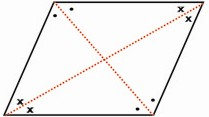HOME MATH DICTIONARY DOWNLOAD FEEDBACK DISCLAIMER
 Question: What is Rhombus ? Answer: A rhombus is a quadrilateral which has its four sides equal in length. It is often described as a diamond because of the way it looks when standing on its end. Opposite sides are parallel and opposite angles are equal. The diagonals bisect each other and are at right angles to one another. The diagonals bisect the interior angles and are lines of symmetry. There is rotational symmetry of order 2 about its center. Example of a Rhombus: